output.to from Sideway
Mechanics: Statics

Draft for Information Only

# Content

`System of Forces Reduction Vector Decomposition Equivalent Systems of Forces Types of Equivalent Systems of Forces`

# System of Forces Reduction

When a force system consists of more than one force, the system of forces should be reduced to one force and one couple. System of forces, F1, F2, and F3 acting on a rigid body at points, A, B, and C, respectively can be transformed to the point of interest, O through force vector transformation,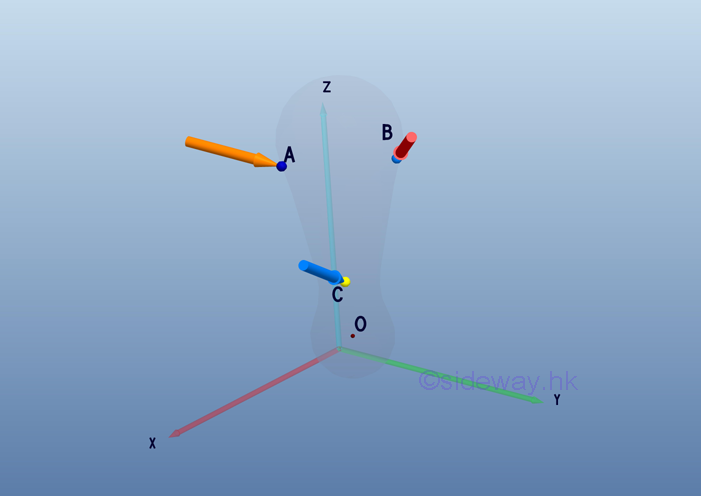According to force vector transformation, forces at different points of application can be moved to the point of interest, O. And the moments of forces about the point of interest, O can also be determined by the cross product of the moment arm of each force vector and the force vector.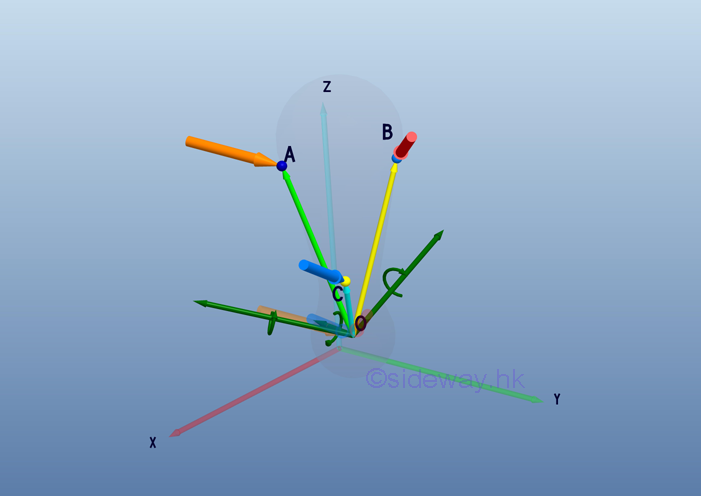After all force vectors are moved to point O and all moment vectors are replaced by couple vectors. The equivalent system acting at a point O on a rigid body can be determined by the vector sum of the all force vectors and the vector sum of all couple vectors at the point O.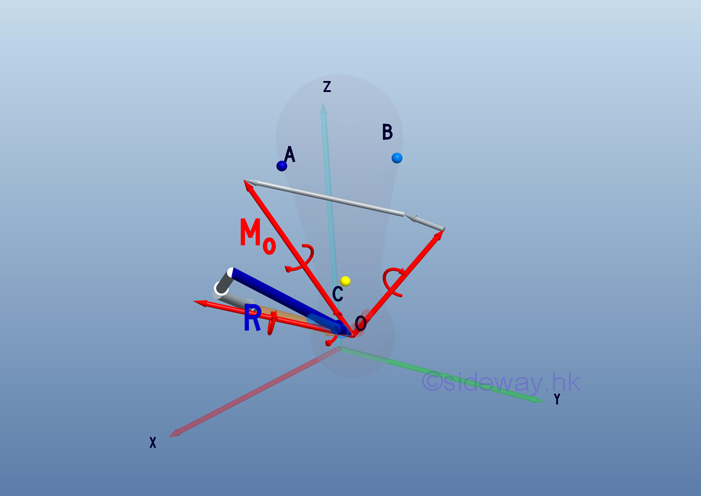Therefore, a system of force can be reduced to an equivalent force-couple system consists of a force vector R and a couple vector MO.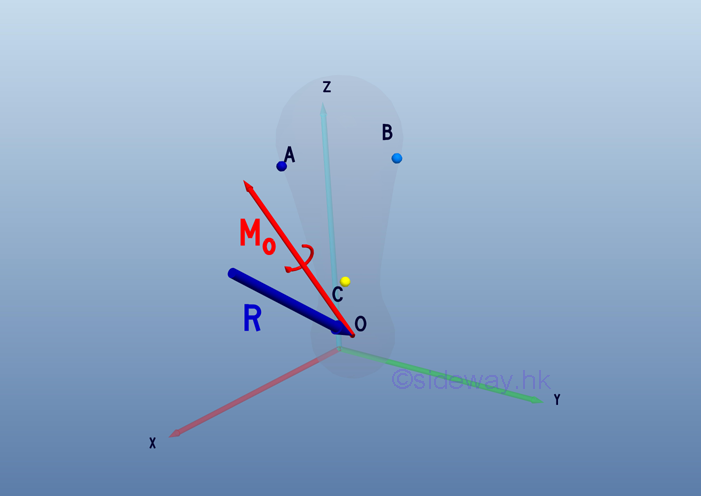Mathematically, the force vector R and the couple vector MO of equivalent system consists can be expressed as: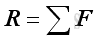and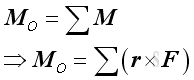The equivalent force-couple system can then be further transferred to other points of interest through the force-couple system transformation.

## Vector Decomposition

The resultant force vector and resultant couple vector can be determined in terms of rectangular compontents through vector decomposition. Imply the force vector and moment arm vector equal to:and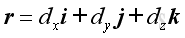And the resultant force vector and couple vector equal to: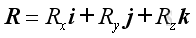and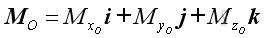The resultant force vector equal to: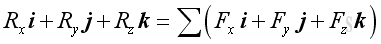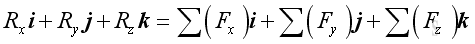Imply: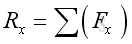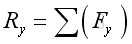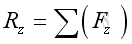The resultant couple vector equal to: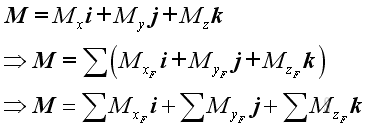.

where:## Equivalent Systems of Forces

Therefore two systems of forces are equivalent at the point of interest, if: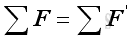and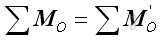Or in terms of rectangular components: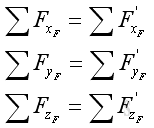and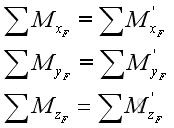## Types of Equivalent Systems of Forces

The two elements of an equivalent force-couple system at O are a resultant force vector R and a resultant couple vector MO at O.

1. When the resultant force vector R equals to zero, the force-couple system becomes a couple vector system.

2.  If the force vector is not equal to zero and the couple vector is normal to the force vector, it can be reduced to a single force system through system of forces transformation.

According to the types of systems of forces, only some typical types of forces system can be reduced to an equivalent force system. They are

1. All concurrent forces systems can always be reduced to a single resultant force vector.
2. All coplanar forces systems can always be reduced to a single resultant force vector lying in the same plane and a couple vector prependicular to that plane. Because the force vector and couple vector are mutually perpendicular, they can be reduced to a single resultant force by moving the  force vector in the plane to creat the moment about the point of interest equal to the couple vector  as in Force-Couple system Reduction.
3. All parallel forces systems can always be reduced to a single resultant force vector parallel to the forces of the system and a couple vector that lying in the plane normal to the force vector. Because the force vector and couple vector are mutually perpendicular, they can be reduced to a single resultant force by moving the  force vector in the plane to creat the moment about the point of interest equal to the couple vector  as in Force-Couple system Reduction.
3. However, if the force vector and couple vector are not matually perpendicular, it remains a force-couple system.

4. But when both the force vector and couple vector are equal to zero, the equivalent system of forces on the rigid body is zero.

ID: 110700014 Last Updated: 2/8/2011 Revision: 1 Ref:References

1. I.C. Jong; B.G. rogers, 1991
2. F.P. Beer; E.R. Johnston,Jr.; E.R. Eisenberg, 2004Home 5

Management

HBR 3

Information

Recreation

Culture

Chinese 1097

English 337

Computer

Hardware 149

Software

Application 187

Numeric 19

Programming

Web 757

CSS 1

HTML

Knowledge Base

OS 389

MS Windows

Knowledge

Mathematics

Algebra 20

Geometry 18

Calculus 67

Engineering

Mechanical

Rigid Bodies

Statics 92

Dynamics 37

Control

Physics

Electric 10